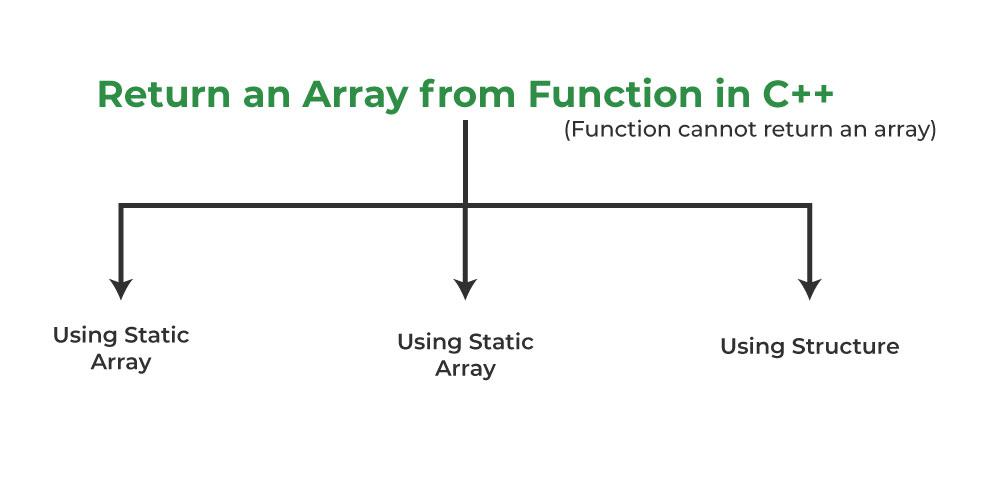Open in App
Not now

# How to Return a Local Array From a C++ Function?

• Difficulty Level : Medium
• Last Updated : 14 Dec, 2022

Here, we will build a C++ program to return a local array from a function. And will come across the right way of returning an array from a function using 3 approaches i.e.

1. Using Dynamically Allocated Array
2. Using Static Array
3. Using Struct

## C++

 `// C++ Program to Return a Local``// Array from a function While``// violating some rules``#include ``using` `namespace` `std;` `int``* fun()``{``    ``int` `arr;` `    ``// Some operations on arr[]``    ``arr = 10;``    ``arr = 20;` `    ``return` `arr;``}` `int` `main()``{``    ``int``* ptr = fun();``    ``cout << ptr << ``" "` `<< ptr;``    ``return` `0;``}`

Warning:

```In function 'int* fun()':
int arr;
^```

The above program is WRONG. It may produce values of 10 or 20 as output or may produce garbage values or may crash. The problem is, that we return the address of a local variable which is not advised as local variables may not exist in memory after the function call is over.

## Following are some correct ways of returning an array## 1. Using Dynamically Allocated Array

Dynamically allocated memory (allocated using new or malloc()) remains there until we delete it using the delete or free(). So we can create a dynamically allocated array and we can delete it once we come out of the function.

Example:

## C++

 `// C++ Program to Demonstrating returning of a local array``// from a function Using Dynamically Allocated Array``#include ``using` `namespace` `std;` `int``* fun()``{``    ``int``* arr = ``new` `int``;` `    ``// Some operations on arr[]``    ``arr = 10;``    ``arr = 20;` `    ``return` `arr;``}` `int` `main()``{``    ``int``* ptr = fun();``    ``cout << ptr << ``" "` `<< ptr;` `    ``// allocated memory must be deleted``    ``delete``[] ptr;``    ``return` `0;``}`

Output

`10 20`

## 2. Using static Array

The lifetime of a static variable is throughout the program. So we can always create a local static array and return it.

Example:

## C++

 `// C++ Program to Demonstrating``// returning of a local array from``// a function Using Static Array``#include ``using` `namespace` `std;` `int``* fun()``{``    ``static` `int` `arr;` `    ``// Some operations on arr[]``    ``arr = 10;``    ``arr = 20;` `    ``return` `arr;``}` `int` `main()``{``    ``int``* ptr = fun();``    ``cout << ptr << ``" "` `<< ptr;``    ``return` `0;``}`

Output

`10 20`

## 3. Using struct

We can wrap the array in a structure/class and return an instance of the struct/class. The reason for this work is, that the array of members of structures is deeply copied. In the below program deep copy happens when we returned instance is copied in main.

Example:

## C++

 `// C++ Program to Demonstrating returning of a local``// array from a function using Struct``#include ``using` `namespace` `std;` `struct` `arrWrap {``    ``int` `arr;``};` `struct` `arrWrap fun()``{``    ``struct` `arrWrap x;` `    ``x.arr = 10;``    ``x.arr = 20;` `    ``return` `x;``}` `int` `main()``{``    ``struct` `arrWrap x = fun();``    ``cout << x.arr << ``" "` `<< x.arr;``    ``return` `0;``}`

Output

`10 20`

My Personal Notes arrow_drop_up